I took part in another CTF, the Icelandic IceCTF. The the competition lasted a whole two weeks from 2015-08-10 to 2015-08-23. I decided to write a writeup of some of the problems I solved.

## 2x0r

We are given file encrypted by the following:

As the xor operation is associative – $$(a \oplus b) \oplus c = a \oplus (b \oplus c)$$, double xor is equivalent to single xor and so it can be solved in the same way. xortool is a very useful tool for finding the key length and then cracking the key.

(the plaintext is utf-8 encoded, so it needs to be manually decoded as some of the characters come out mangled in the xortool-produced result)

Note that if the keys have lengths $$a,\space b$$ the equivalent single key length will be $$lcm(a, b)$$ bytes, which can be significantly larger than either $$a$$ or $$b$$. In this case this corresponds to $$lcm(7, 9) = 63$$.

## Hackers in disguise

So, it turns out, that perl’s open() has this fascinating “feature” allowing for command injection in the string passed to the call.

## Fermat

Variadic function calls in C do not pass the number of parameters actually given to the function being called. This means that the callee has to derive it by some other means. In case of printf and the like it is by the amount of flags passed in the format string. An attacker in control of the string can then make printf to read data further down the stack which are not arguments. e. g. to dump the stack content:

The address of the secret variable is 0x804a02c, which corresponds to the sixth argument. Now we need to write to it. One of the printf flag characters is %n, which writes the amount of characters already printed into a pointer. This allows the attacker to write (well, almost) arbitrary values to any pointer on the stack. Using that, the exploit string looks like this.

## RSA3

This time the provided file contains only the public key, the exponent and the ciphertext. As the hint says that the message is short and the public exponent $$e = 3$$ the ciphertext is easy to recover.

RSA encryption is defined as

$c = m^e \bmod N$

From this follows that:

$m = \sqrt[e]{c + kN}$

By inspection, it can be seen, that if $$m$$ is small, then $$k$$ will also be small. Therefore it can easily be bruteforced:

## Barista

In this problem, the target is a web service written in CoffeeScript

This one took me quite a long time… The point here is, that JS objects are not maps. In a standard map, one would not expect any keys to be there by default.

Therefore we can get the application to call an unexpected function like:

Notice that all is_admin checks are not strictly typed, so it does not matter what does the getter return as long as it evaluates to true.

## PyShell

This looks kind of bulletproof doesn’t it? Well, with some python trickery, the sandbox can be broken and the flag captured.

## Entropy

This is a reference to the famous Debian key generation bug. After enough keys are gathered, some of them are going to have at least one prime in common with the key with which the message was encrypted. If two numbers share a prime factor, it can be recovered easily using Euclid’s algorithm and then the other prime factor can be recovered just by division.

## Authorize

SQL Injection here. As there is no easy way to read the database directly, so I just opted to use sqlmap which can dump the database by using blind SQLI.

## Agents

At first, this looked like the Håstad’s Broadcast Attack, but after implementing it in its vanilla form, it didn’t work. The hint seems to hint (hints in IceCTF are somewhat cryptic…) that motd.txt is longer than the public modulus. Remember, RSA can only encrypt $$m < n$$.

What to do now? Let’s look at what Chinese Remainder Theorem gives us.

Given a system of equations of the form:

$c \equiv m^e \bmod n_1 \\ c \equiv m^e \bmod n_2 \\ ... \\ c \equiv m^e \bmod n_I$

It calculates:

$r = c \bmod N \\ \text{where} \space N = n_1 n_2 ... n_I$

In the standard broadcast attack, it is guaranteed that $$c < N$$ as soon as $$I >= e$$, because $$m^e < min(n)^e$$. Here however, is no such guarantee. So the only thing left is to gather more keys and ciphertexts and see how many we need to get an integer $$e-th$$ root.

This is actually quite cool. Every individual RSA encryption loses some information, but when enough results are gathered, the plaintext can be quite elegantly recovered.

## Mondrian

This video is highly reminiscent of the Webdriver Torso YouTube channel. First, let’s take a look at the words being said.

Concatenating first letter of each word forms webdriver. Now, overlaying each pair of rectangles with a crude python script leads to:Nothing exactly surprising yet. There is an html comment on the page containing the video: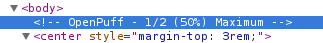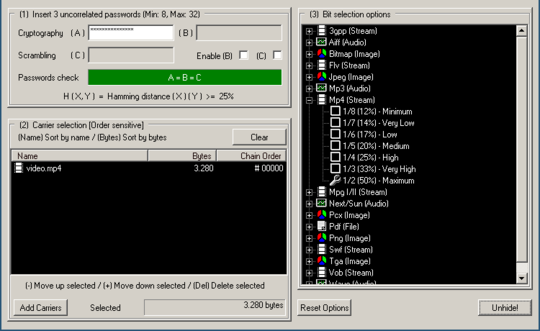Which leads to OpenPuff, steganography software, sadly for Windows. Luckily though, it runs under Wine just fine. After setting it according to the comment and using the password webdriver torso, it spits out mondrian.bmp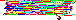This image is actually a program, “written” in an esoteric language called piet. After picking an interpreter and running the image, it printed the flag to stdout.

## SuperNote

So, the objective is to write a python script into the cron.d directory. The note is saved into the temporary file (created by tempnam()). The trick is, to make a symlink pointing from the temporary file into cron.d so the application writes content of the note there. The files inside cron.d are just python scripts with .task suffix.

## Wiki & The Furious

This time, there is no persistent XSS. However, there is a comment functionality and there is a “Report Abuse” button for each individual button.The permalink button generates a link with URL fragment of the form:

The Report Abuse sends a POST request containing:Hm, so it seems that we can inject arbitrary fragment into the URL the admin opens. Let’s take a look at how the fragment is processed.

Because jQuery selector does not only select elements but is also able to create them, this code is vulnerable to DOM XSS. The exploit url then looks like:

## What

This is a first binary exploitation challenge which does not have sources available.

So, let’s open radare2 and see what it does.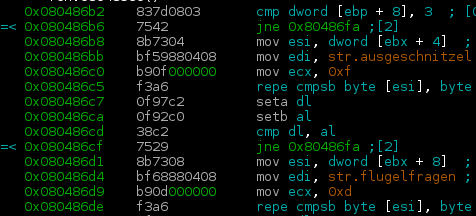First, it takes the first two args and compares them to ausgeschnitzel and flugelfragen (I have no idea what these words mean and google translate is silent too).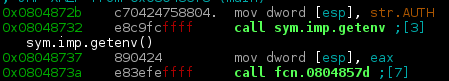It then gets the AUTH environment variable and passes it to a function

What happens when AUTH is empty?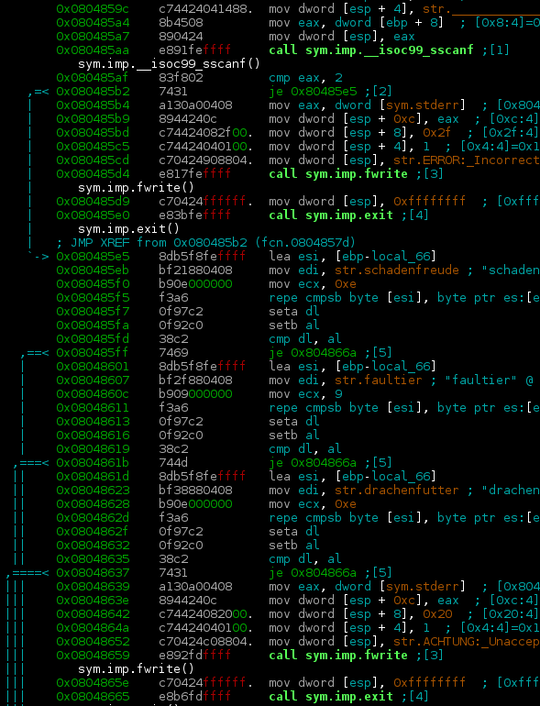There is an obvious buffer overflow in both the variables containing WHO and WHY. As WHY has to contain one of the valid reason strings, otherwise exit() gets called, this leaves us with WHO to exploit. The tricky part here is, that the exploit cannot contain / (0x2f). This means that my favourite x86 shellcode cannot be used. Metasploit to the rescue!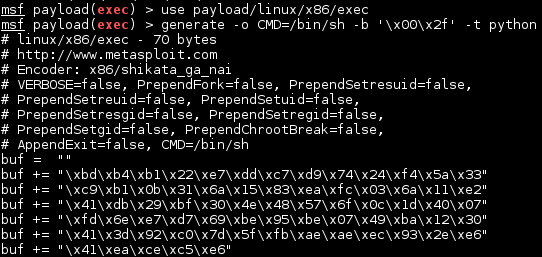## Giga

Objective here is to get the application to unserialize new File("flag.txt") as it outputs its contents in its destructor if ?debug=1 is set.

The fact that using $$hash(secret + message)$$ to sign messages instead of HMAC is bad idea is well known as it can be vulnerable to the Length extension attack which, given $$hash(secret + s_1), \space s_1$$, can compute $$hash(secret + s_2)$$ (the attacker has to know the length of secret, which in this case is 40 bytes, but it can be easily brute forced if unknown).

We have the value of hash("sha256", AUTH_SECRET . strrev(serialize(false))), and need hash("sha256", AUTH_SECRET . strrev(serialize(new File("flag.txt")))) Luckily, there is a Python library hlextend which implements the necessary functions to calculate the hash.

## Conclusions

This week I learned…

• RSA is really trivial to break without proper padding
• jQuery’s \$(…) needs to have sanitized input
• Perl is weird
• Metasploit is able to generate shellcode without specific arbitrary characters
• There is an easy way to exploit $$hash(secret + message)$$ used as signature
• JavaScript objects can behave unexpectedly when used as a map
• piet exists
• sqlmap is awesome